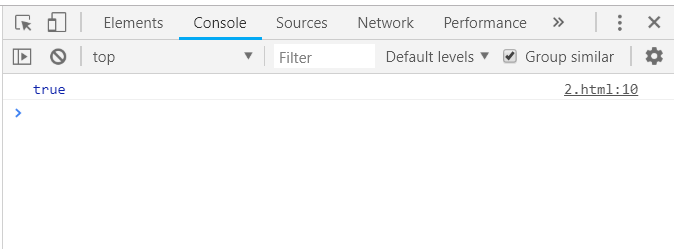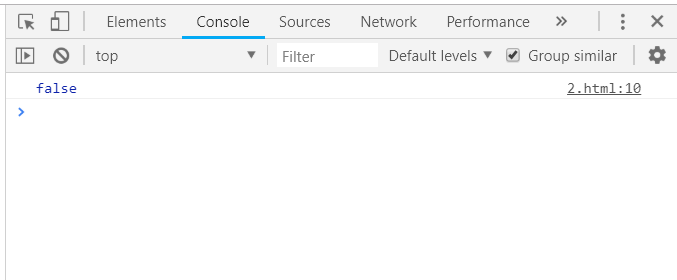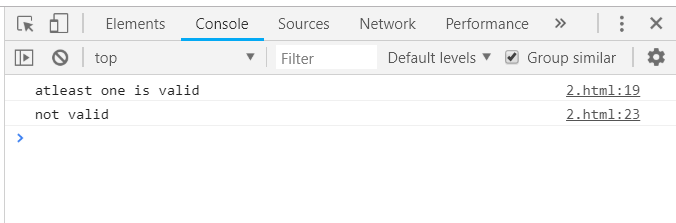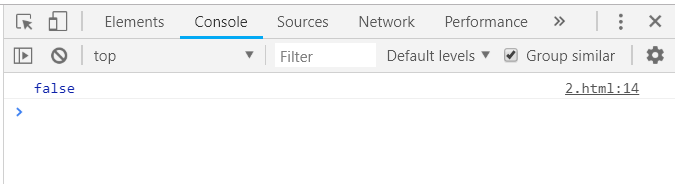# Underscore.js | some() with Examples

The Underscore.js is a JavaScript library that provides a lot of useful functions that helps in the programming in a big way like the map, filter, invoke etc even without using any built-in objects.
The _.some() function is used to find whether any value in the given list matches the given condition or not. If at least one value satisfies this condition then the output will be true. When none of the values matches then the output will be false

Syntax:

```_.some(list, [predicate], [context])
```

Parameters: This function accepts three parameters as mentioned above and described below:

• List: This parameter contains the list of data.
• Predicate: This parameter is used to hold the test condition.
• Context: This parameter contains the text which need to display.

Return values: The return value which is either true (when at least one element of the list fulfills the given condition) or false (when none of the elements fulfill the condition).

Passing an array to the _.some() function(): The ._some() function takes the element from the list one by one and then check the condition by doing the specified operations on the code. The operation is to find whether the array contains any true elements or not.

Example:

## HTML

 `<``html``>` `    ``<``head``>` `        ``<``title``>_.some() function` `        ``<``script` `type``=``"text/javascript"` `src``=` `        ``"https://cdnjs.cloudflare.com/ajax/libs/underscore.js/1.9.1/underscore-min.js"` `>` `        ``` `        ``<``script` `type``=``"text/javascript"` `src``=` `        ``"https://cdnjs.cloudflare.com/ajax/libs/underscore.js/1.9.1/underscore.js"``>` `        ``` `    ``       ` `    ``<``body``>` `        ``<``script` `type``=``"text/javascript"``>` `              ``console.log(_.some([null, 0, 1, false]));` `        ``` `    ``` ``

Output:In the below code since the array contains all false elements like ‘0’, ‘false’, ‘null’ and no true elements, so the output will be ‘false’.

Example:

## HTML

 `<``html``>` `    ``<``head``>` `        ``<``title``>_.some() function` `        ``<``script` `type``=``"text/javascript"` `src``=` `        ``"https://cdnjs.cloudflare.com/ajax/libs/underscore.js/1.9.1/underscore-min.js"` `>` `        ``` `        ``<``script` `type``=``"text/javascript"` `src``=` `        ``"https://cdnjs.cloudflare.com/ajax/libs/underscore.js/1.9.1/underscore.js"``>` `        ``` `    ``       ` `    ``<``body``>` `        ``<``script` `type``=``"text/javascript"``>` `             ``console.log(_.some([null, 0, false]));` `        ``` `    ``` ``

Output:Passing a list of numbers and a function to _.some() function: First, define both list and the function which need to performed/checked on the list. Then pass the list and the function as arguments to the _.some() function. If the condition given in the function is satisfied even by 1 of the element of the list then the output will be true.

Example:

## HTML

 `` `<``html``>` ` `  `<``head``>` `    ``<``script` `type``=``"text/javascript"` `src` `= ``"https://cdnjs.cloudflare.com/ajax/libs/underscore.js/1.9.1/underscore-min.js"` `>` `    ``` `` ` `  `<``body``>` `    ``<``script` `type``=``"text/javascript"``>` `         ``var values = [1, 2, 3, 4, 5, 6, 7, 8, 9, 10];` `         ``var isEven = function (value) {` `               ``return value % 2 === 0;` `        ``};` `        ``console.log(_.some(values, isEven));` `    ``` `` ` `  ``

Output:Passing a structure to the _.some() function: First declare the array (here array is ‘people’). Choose one condition on which need to check like here ‘longHairs’. Console.log the final answer. Since, three people’s ‘longHair’ property is true so the resultant is also true.

Example:

## HTML

 `<``html``>` `    ``<``head``>` `        ``<``title``>_.some() function` `        ``<``script` `type``=``"text/javascript"` `src``=` `        ``"https://cdnjs.cloudflare.com/ajax/libs/underscore.js/1.9.1/underscore-min.js"` `>` `        ``` `        ``<``script` `type``=``"text/javascript"` `src``=` `        ``"https://cdnjs.cloudflare.com/ajax/libs/underscore.js/1.9.1/underscore.js"``>` `        ``` `    ``       ` `    ``<``body``>` `        ``<``script` `type``=``"text/javascript"``>` `             ``var people = [` `                ``{name: 'sakshi', LongHair: ''},` `                ``{name: 'aishwarya', LongHair: true},` `                ``{name: 'akansha', LongHair: true},` `                ``{name: 'preeti', LongHair: true}` `            ``],` `      `  `            ``hasLongHairs = function (value) {` `                ``return (value.LongHair !== '');` `            ``};` `     `  `            ``console.log(_.some(people, hasLongHairs));` `        ``` `    ``` ``

Output:Using two _.some() function together: Passing different objects to each _.some() function and then use the following results together by using the logical operators like ‘&&’, ‘||’, ‘!’ etc. The object1 and arralist1 contains atleast one true value so the resultant of two true will also be true. Hence, first condition is satisfied. The object2 is empty and arraylist2 also is also empty so they are not valid. Since use ‘!’ before every _.some() function so the resultant are 2 true values.

Example:

## HTML

 `<``html``>` `    ``<``head``>` `        ``<``title``>_.some() function` `        ``<``script` `type``=``"text/javascript"` `src``=` `        ``"https://cdnjs.cloudflare.com/ajax/libs/underscore.js/1.9.1/underscore-min.js"` `>` `        ``` `        ``<``script` `type``=``"text/javascript"` `src``=` `        ``"https://cdnjs.cloudflare.com/ajax/libs/underscore.js/1.9.1/underscore.js"``>` `        ``` `    ``       ` `    ``<``body``>` `        ``<``script` `type``=``"text/javascript"``>` `            ``var listOne = [null,, undefined, {}, 0];` `            ``var listTwo = [];` `            ``var objectOne = {` `                ``property1: null,` `                ``property3: true` `            ``};` `            ``var objectTwo = {};` `            ``if (_.some(listOne) && _.some(objectOne)) ` `            ``{` `                ``console.log("atleast one is valid\n")` `            ``};` `            ``if (!_.some(listTwo) && !_.some(objectTwo)) ` `            ``{` `                ``console.log("not valid\n")` `            ``};` `        ``` `    ``` ``

Output:Example:

## HTML

 `<``html``>` `    ``<``head``>` `        ``<``title``>_.some() function` `        ``<``script` `type``=``"text/javascript"` `src``=` `        ``"https://cdnjs.cloudflare.com/ajax/libs/underscore.js/1.9.1/underscore-min.js"` `>` `        ``` `        ``<``script` `type``=``"text/javascript"` `src``=` `        ``"https://cdnjs.cloudflare.com/ajax/libs/underscore.js/1.9.1/underscore.js"``>` `        ``` `    ``       ` `    ``<``body``>` `        ``<``script` `type``=``"text/javascript"``>` `            ``var values = [1, 3, 5, 7, 9];` `             ``var isEven = function (value) {` `                   ``return value % 2 === 0;` `            ``};` `            ``console.log(_.some(values, isEven));` `        ``` `    ``` ``

Output:My Personal Notes arrow_drop_upI like to do coding in C++C and java programming languages HTML and CSS always intersts me Sharing knowleged is the best way according to me to increase ones knwoledge

If you like GeeksforGeeks and would like to contribute, you can also write an article using contribute.geeksforgeeks.org or mail your article to contribute@geeksforgeeks.org. See your article appearing on the GeeksforGeeks main page and help other Geeks.

Please Improve this article if you find anything incorrect by clicking on the "Improve Article" button below.

Improved By : arorakashish0911